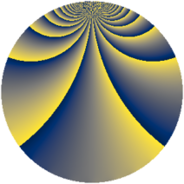# Properties

 Label 1600.2.bjLevel $1600$ Weight $2$ Character orbit 1600.bj Rep. character $\chi_{1600}(161,\cdot)$ Character field $\Q(\zeta_{10})$ Dimension $240$ Sturm bound $480$

# Related objects

## Defining parameters

 Level: $$N$$ $$=$$ $$1600 = 2^{6} \cdot 5^{2}$$ Weight: $$k$$ $$=$$ $$2$$ Character orbit: $$[\chi]$$ $$=$$ 1600.bj (of order $$10$$ and degree $$4$$) Character conductor: $$\operatorname{cond}(\chi)$$ $$=$$ $$200$$ Character field: $$\Q(\zeta_{10})$$ Sturm bound: $$480$$

## Dimensions

The following table gives the dimensions of various subspaces of $$M_{2}(1600, [\chi])$$.

Total New Old
Modular forms 1008 240 768
Cusp forms 912 240 672
Eisenstein series 96 0 96

## Trace form

 $$240q + 60q^{9} + O(q^{10})$$ $$240q + 60q^{9} + 24q^{17} - 12q^{25} - 24q^{41} + 240q^{49} + 60q^{65} + 24q^{73} - 60q^{81} - 36q^{89} + 168q^{97} + O(q^{100})$$

## Decomposition of $$S_{2}^{\mathrm{new}}(1600, [\chi])$$ into newform subspaces

The newforms in this space have not yet been added to the LMFDB.

## Decomposition of $$S_{2}^{\mathrm{old}}(1600, [\chi])$$ into lower level spaces

$$S_{2}^{\mathrm{old}}(1600, [\chi]) \cong$$ $$S_{2}^{\mathrm{new}}(200, [\chi])$$$$^{\oplus 4}$$$$\oplus$$$$S_{2}^{\mathrm{new}}(800, [\chi])$$$$^{\oplus 2}$$